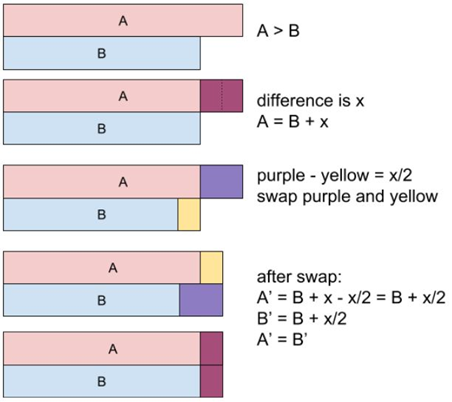# Fair Candy Swap

## Introduction

Candy swap is a problem of Hashing and Array where we have to find the amount of candies we need to swap between two friends to make the total amount between them the same. Concepts related to Array and Hashing should be clear in your head if you have to master Data Structures and Algorithms

We will see different approaches from brute force to an efficient one, which will help you build the logic more easily.

Without any delays, let's move to our problem statement.

## Problem Statement

There are two friends Alice and Bob, and both are very fond of candies. They have a different total number of candies. You are given two integer arrays, of which one array contains the total number of candies that Alice has, and another array contains the total number of candies that Bob has.

As they are best friends, they would like to exchange one candy box each so that after the exchange, they both have the same number of candies. The total number of candies a person has is the sum of candies in each box.

We have to return the array, where the first index of the array will contain the number of candies that Alice must exchange, and the second index of the array will include the number of candies that Bob must exchange.

Note:

• There can be multiple answers; return any one of them.
• At least one answer will be there.

Let me make you more precise with the help of an example.

Input

Alice = [1,1] , Bob = [2,2]

Output:

[1,2]

In this example, Alice has in total two candies(1+1=2), and Bob has in total four candies(2+2=4), which is not the same. To make the total number of candies the same, we have to swap Alice and Bob.

If we swap Alice having candy at index=0 and Bob having candy at index=0, then Alice will have three candies in total, and Bob will also have three candies in total, which is the same amount.

After swapping the arrays will become:

Alice = [2,1] , Bob = [1,2]

Therefore, the result array will be [1,2].

If the problem statement is clear, then let's move towards the approach.

Building of Approach

The building of the logic will be clear through this pictorial representation.A = Alice

B = Bob

Therefore, our target is to find a candy pair whose difference is exactly x/2.

If B > A, logic is precisely the same.

## Approach 1: Brute Force

In the brute force approach, we will be using nested loops to calculate the difference between Alice's and Bob's candies.

Algorithm

• We will create two variables, namely Sum1 and Sum2, and initialize them with '0'.
• These variables will store the total number of candies of Alice and Bob.
• We will find the candy pair whose difference is exactly (Sum1 - Sum2)/2.
• Return the candy pair.

## Analysis of Complexity

Time Complexity: Finding an array containing candies that need to be swapped is O(A+B+A*B). Where A is for Alice and B is for Bob.

Space Complexity: The space complexity will be O(1). No extra space is needed.

## Approach 2: Efficient

Another approach that we will see is HashSet. HashSet stores elements by using hashing. It contains unique elements only and allows null values. We are using HashSet to improve our time complexity as it stores unique box sizes.

## Analysis of Complexity

Time Complexity: O(A + B) where A = Alice and B = Bob.

Space Complexity: O(A)

1. What is HashSet?

HashSet stores unique elements by using the mechanism called Hashing. It allows storing null values in a non-synchronized manner.

2. What are the other approaches to find the number of candies to be exchanged?

The other approach to solve this problem is Binary Search. It will take O(nlogn) time

and O(1) space.

3. Why is the time complexity of the HashSet approach O(A + B)?

O(A): first for iterating elements of A

O(B): second and third for iterating elements of B

## Key Takeaways

In this article, we discussed the Candy Swap problem and how we can build an approach for it:

The first approach is the brute force which uses nested loops to find the candy pair. We have to see whether the target(A - B) is equal to (A - B)/2 or not.

The second approach uses HashSet. So that the unique size of boxes can be stored and problems can be solved efficiently.

You can use CodeStudio to practice various DSA questions typically asked in interviews. It will help you in mastering efficient coding techniques.

Keep Coding!!!